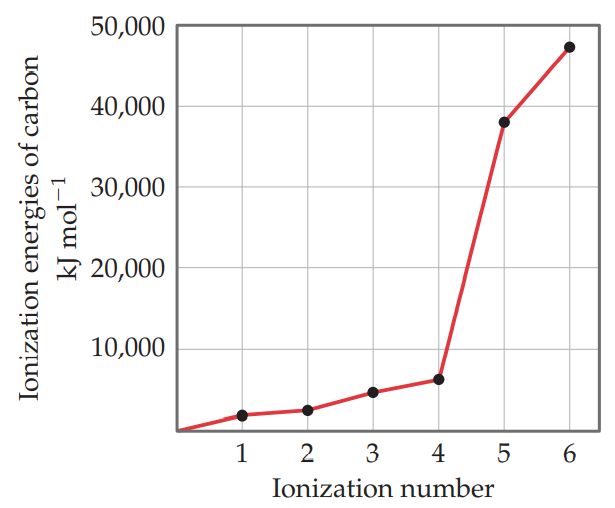×
Get Full Access to Chemistry: The Central Science - 12 Edition - Chapter 7 - Problem 93ae
Get Full Access to Chemistry: The Central Science - 12 Edition - Chapter 7 - Problem 93ae

×

# Explain the variation in the ionization energies ofISBN: 9780321696724 27

## Solution for problem 93AE Chapter 7

Chemistry: The Central Science | 12th Edition

• Textbook Solutions
• 2901 Step-by-step solutions solved by professors and subject experts
• Get 24/7 help from StudySoup virtual teaching assistantsChemistry: The Central Science | 12th Edition

4 5 1 432 Reviews
29
4
Problem 93AE

Explain the variation in ionization energies of carbon, as displayed in this graph:Step-by-Step Solution:
Step 1 of 3

Lecture 08/30/17 → Chapter 2: Graphs and Descriptive Statistics ← Listed Form: 3,4,7,9,10,10,10,14,15,17,17,19,20,20,20 Grouped (Frequency) Form: 3 | 1 4 | 1 7 | 1 9 | 1 10 | 3 14 | 1 15 | 1 17 | 2 19 | 1 20 | 3 ———— 15 Interval (Frequency) Form: Class: 3-9 10-16 17-23 LCL - UCL Lower Class Limit - Upper Class Limit LCB = LCL - tol/2 UCB = UCL + tol/2 Class Width: Length + 1 of the class so 7-7-7 Class Midpoint = (UCL + LCL)/2 9+3 = 12/2 = 6 10 + 16 = 26/2 = 13 17 + 23 = 40/2 = 20 Frequency: 4 5 6 —— 15 Sigma = Sum Ef = 15 = n (Sample Size) Tolerance = LCL of next class - UCL of previous class. Relative frequency = F/total f Symmetric = Bell-shaped Left-Shewed = left bell Right-Shewed = right bell Outliers = data that don’t act like the rest of the data, or not part of it. Histogram Frequency Polygon: Frequency Midpoint Section 2.1 EDA  Exploratory Data Analysis (EDA) is a critical first step in analyzing the data from an experiment. Mostly a graphical approach.  The four types of EDA are: o Univariate non-graphical o Multivariate non-graphical o Univariate graphical, and o Multivariate graphical.  The main reasons we use EDA are: o To get an in depth insight of the data set. o To understand the behavior of the data. o To detect the mistakes/outliers that exists in the dataset. o To check the assumptions. o To extract important attributing variables. o To

Step 2 of 3

Step 3 of 3

## Discover and learn what students are asking

Statistics: Informed Decisions Using Data : Properties of the Normal Distribution
?In Problems 25–28, the graph of a normal curve is given. Use the graph to identify the values of ? and ?.

Unlock Textbook Solution

Enter your email below to unlock your verified solution to:

Explain the variation in the ionization energies of